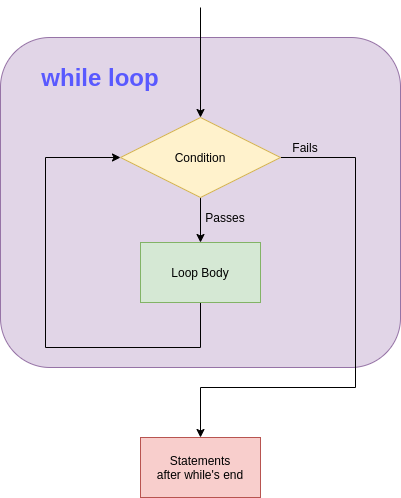No matter what modern programming language you take, you will find a while loop in it, Julia is no exception in that case (but there are languages without it). This blog is about while loops in Julia. The notebook for this blog can be found here: https://gitlab.com/data-science-with-julia/code/-/blob/master/while.ipynb. So let’s try it out and see. Take this first example, type it in your Jupyter lab and execute it:

i = 1

while i <= 10
println(i)
i += 1
end


Output:

1
2
3
4
5
6
7
8
9
10


So how it works? First we have got this statement i = 1, where we initialize a variable named i to 1. Next we have while i <= 10, so this while key word gets a condition i <= 10, and i is less than 10, so the stuff between while <condition> and end gets executed. So in the the above code they are these lines:

println(i)
i += 1


Here we print i in println(i) and next we increment i by 1 here i += 1 and hence i becomes 2. When it encounters end, it does not end, the control transfers back to while i <= 10, since the condition is true again the loop body gets executed again and 2 gets printed and i become 3 now. It goes on till i is 11 and when it hits while i <= 10, i <= 10 fails and the loop never gets executed and the program ends.

For a visual look here is a diagram that explains how a while loop works:A while or most programming loops needs the three things to work properly

1. A variable initiation, for us its i = 1.
2. A condition check, for us its i <= 10.
3. Variable update, for us its i += 1.

If we leave out (3), the loop could be a infinite loop, comment out i += 1 and see what happens, hope you know to stop your kernel and kill jupyter notebook / lab, refer Installing Jupyter notebook and Jupyter lab.